# RD Sharma Solutions for Class 11 Maths Chapter 4 Measurement of Angles

## RD Sharma Solutions Class 11 Maths Chapter 4 – Free PDF Download

RD Sharma Solutions for Class 11 Maths Chapter 4 – Measurement of Angles is provided here. We know that Trigonometry is a branch of Mathematics which deals with the measurement of the sides and the angles of a triangle and the problems allied with angles. In this chapter, we mostly learn about the relation between degrees, radians and real numbers. Expert tutors at BYJU’S have designed the RD Sharma Class 11 Solutions in order to help students to gain more knowledge and create a strong grip over the subject. Students are advised to practice the solutions regularly so that they can come out with flying colours in their exams. Solutions to this chapter are provided in the PDF format, which can be downloaded easily from the links provided below.

RD Sharma Solutions for Class 11 Maths Chapter 4 – Measurement of Angles contains one exercise and the RD Sharma Solutions present in this page provide solutions to the questions present in this exercise. Now, let us have a look at the concepts discussed in this chapter.

• Angles.
• Some useful terms.
• Systems of measurement of angles.
• Sexagesimal system.
• Centesimal system.
• A circular system.
• The relation between degrees and radians.
• The relation between radians and real numbers.
• The relation between three systems of measurement of an angle.

## Download the Pdf of RD Sharma Solutions for Class 11 Maths Chapter 4 – Measurement of Angles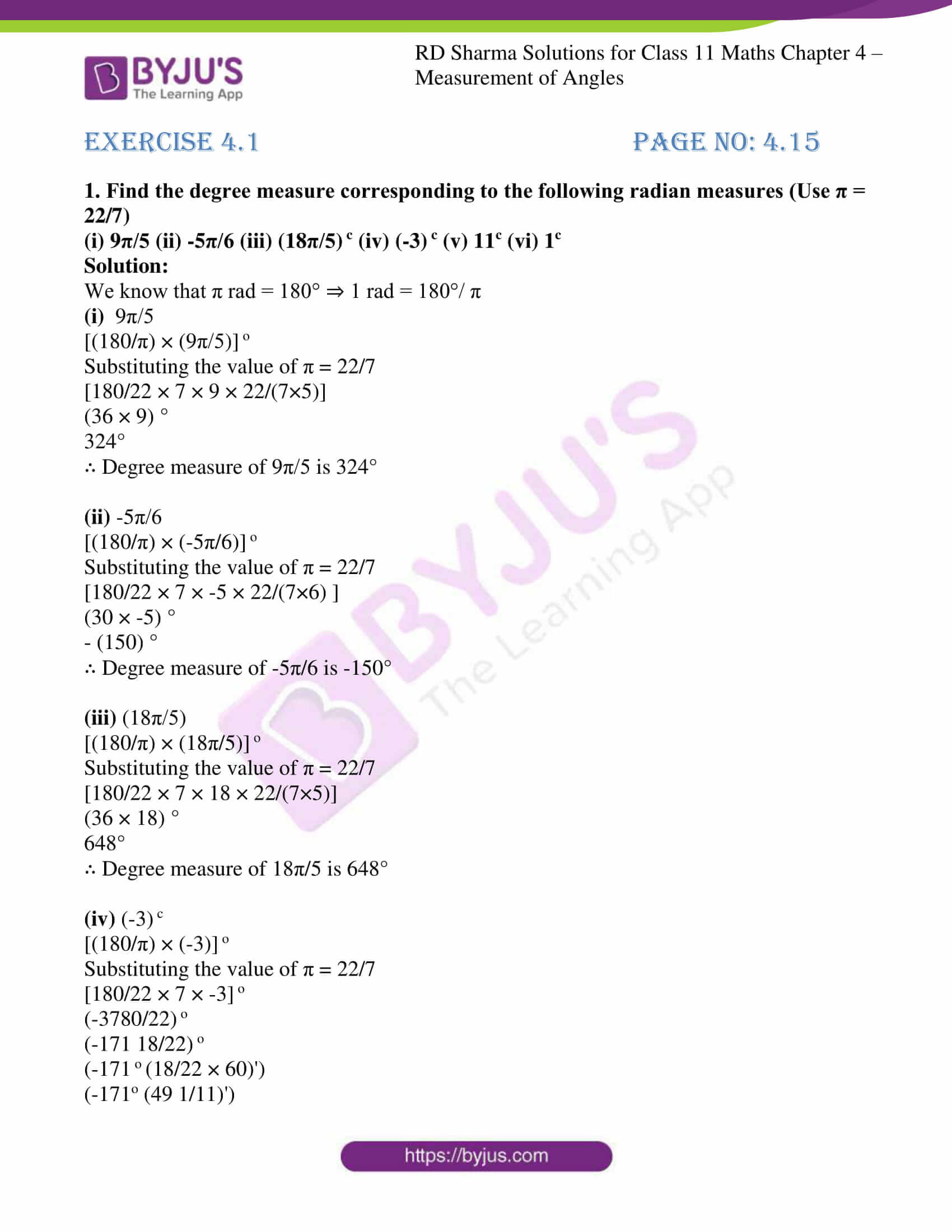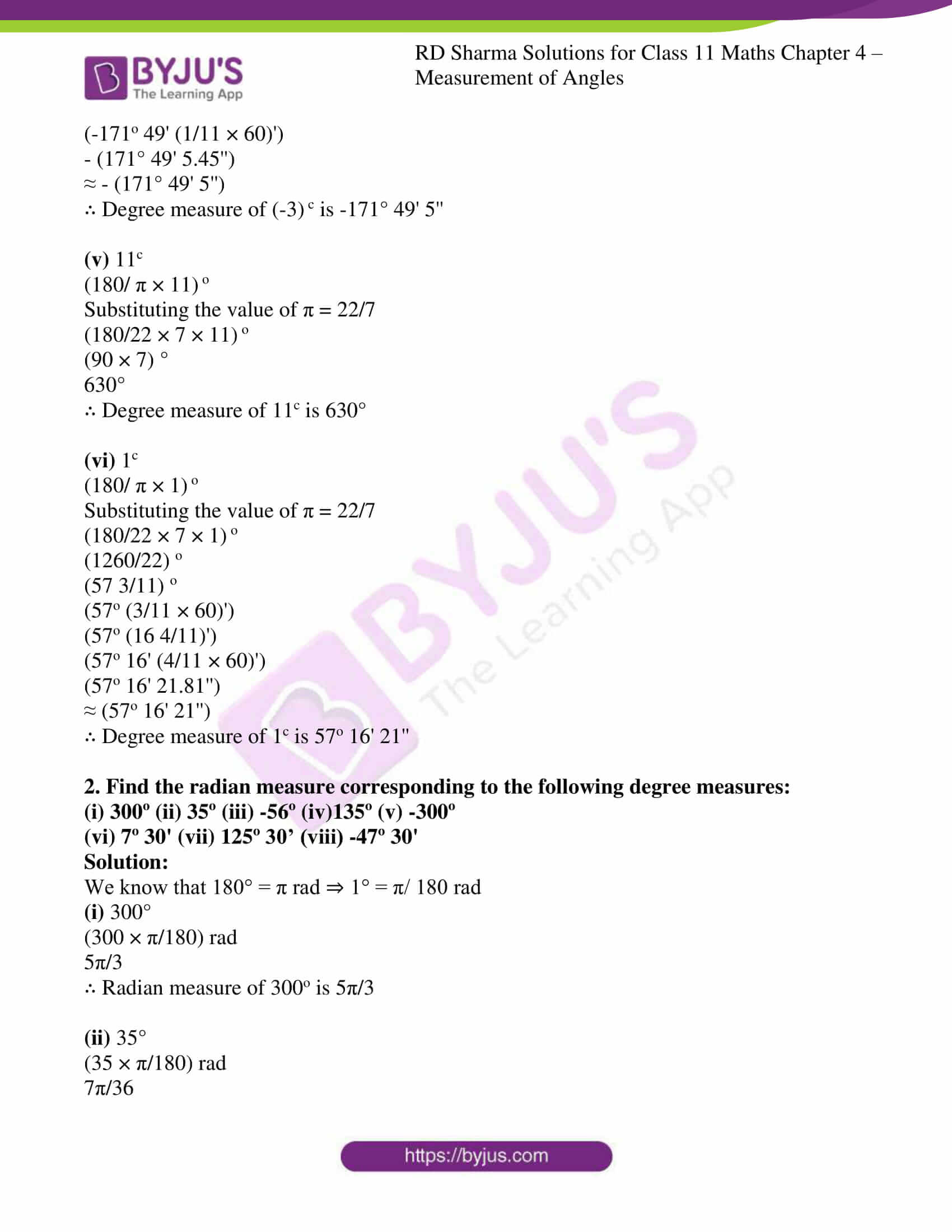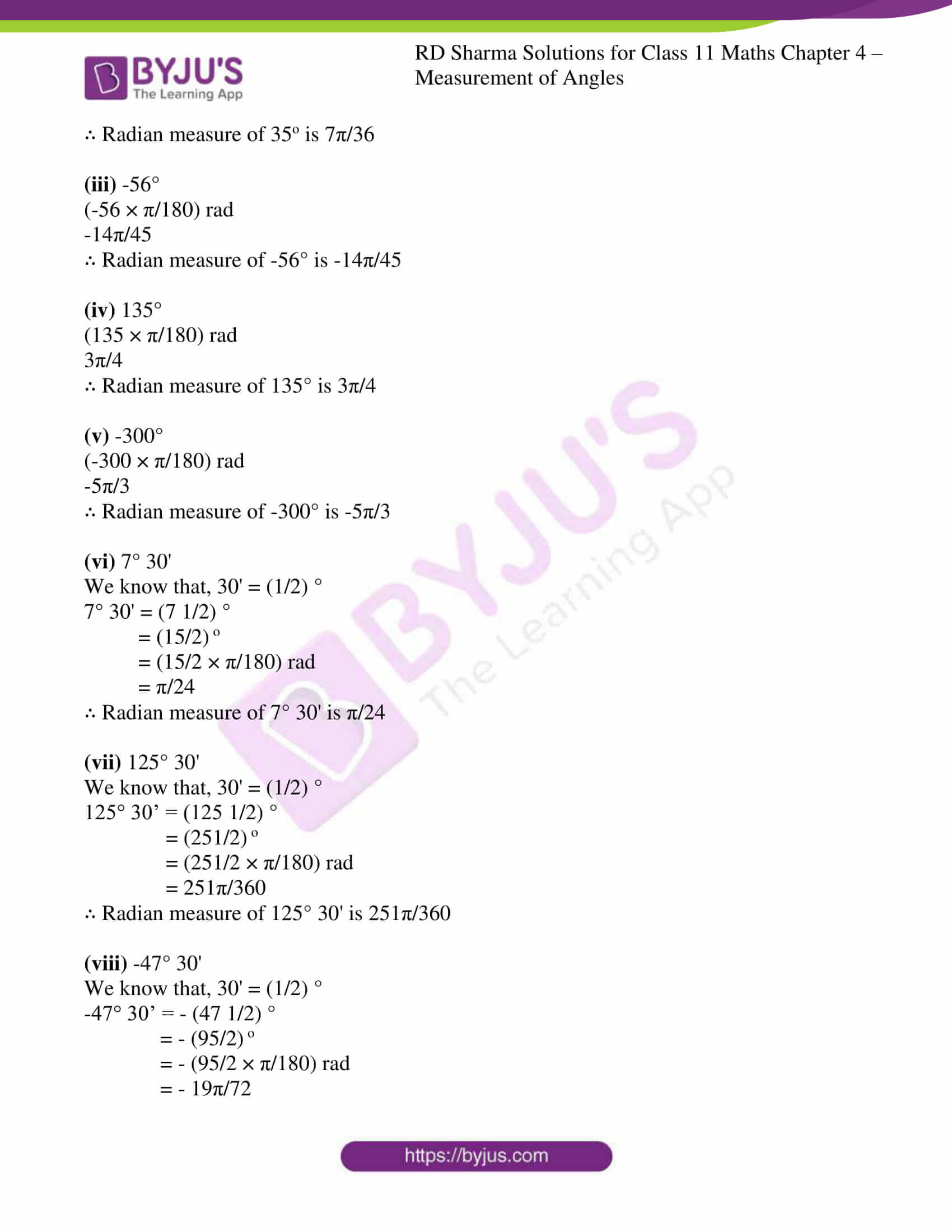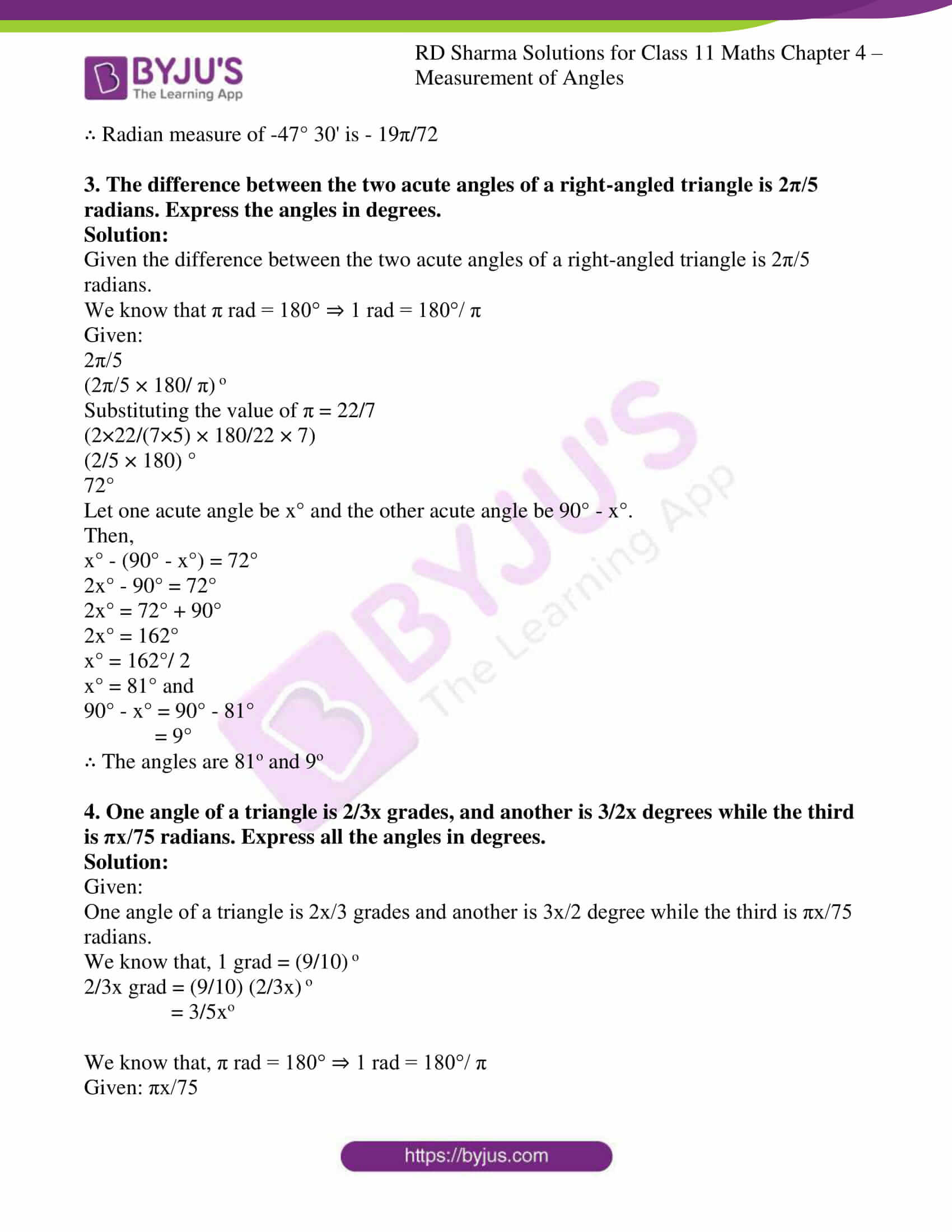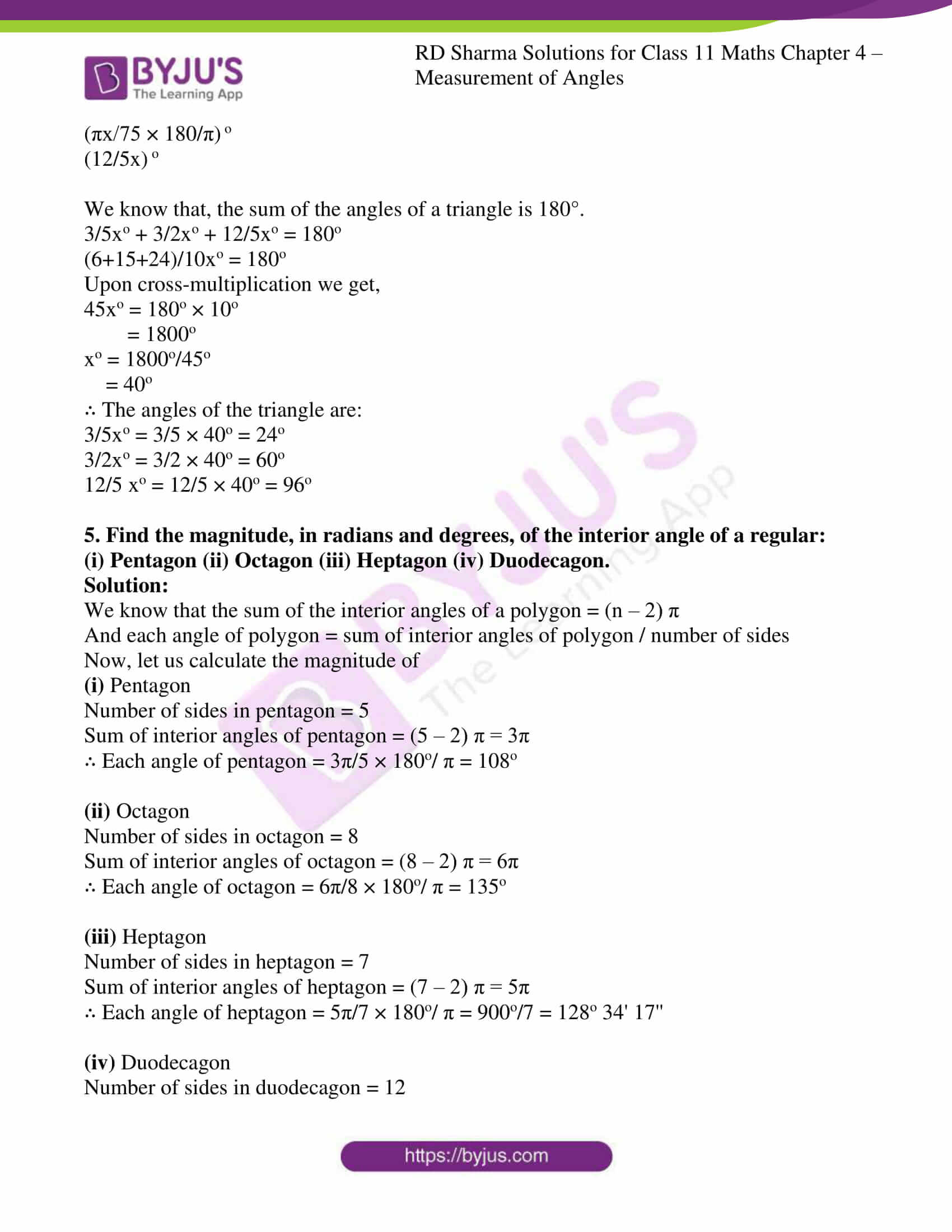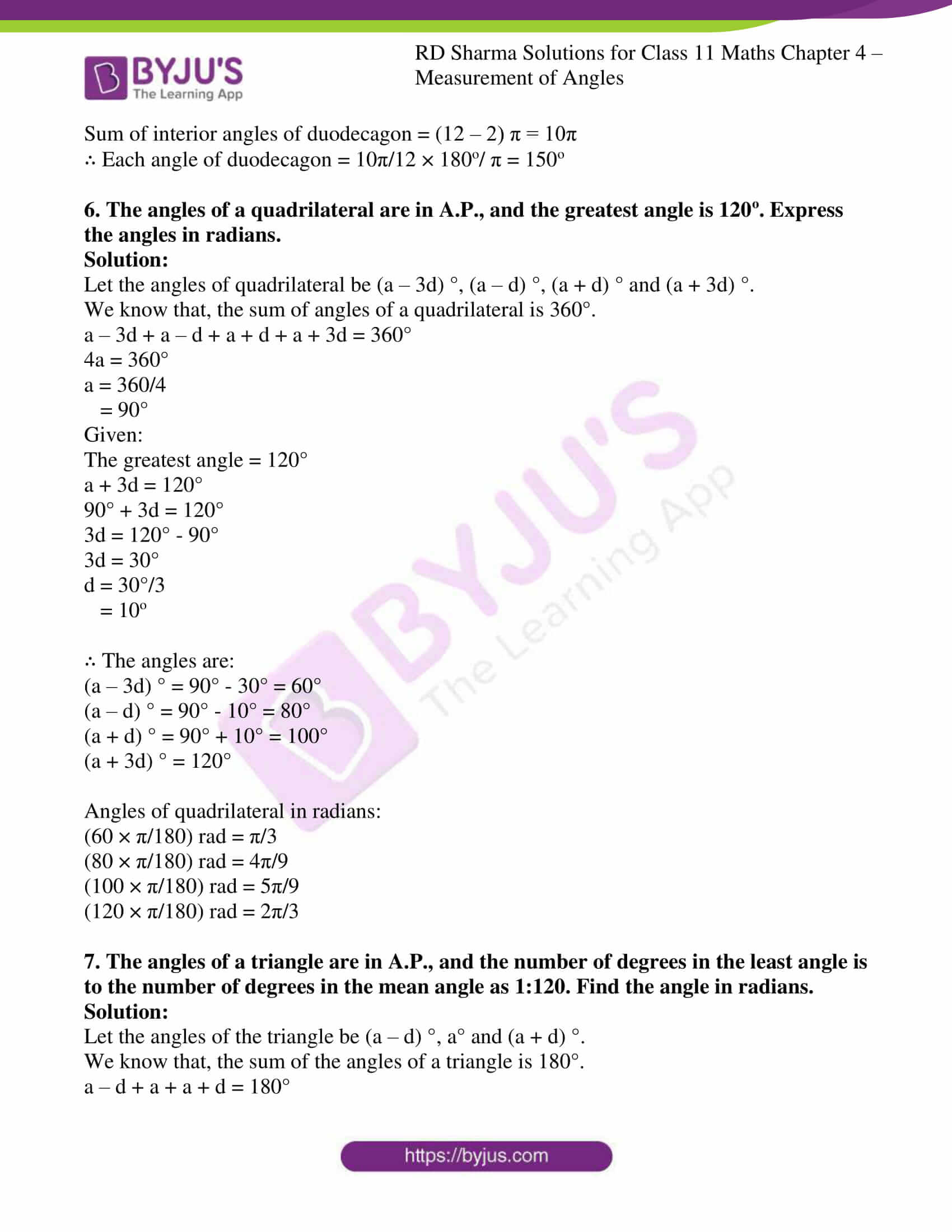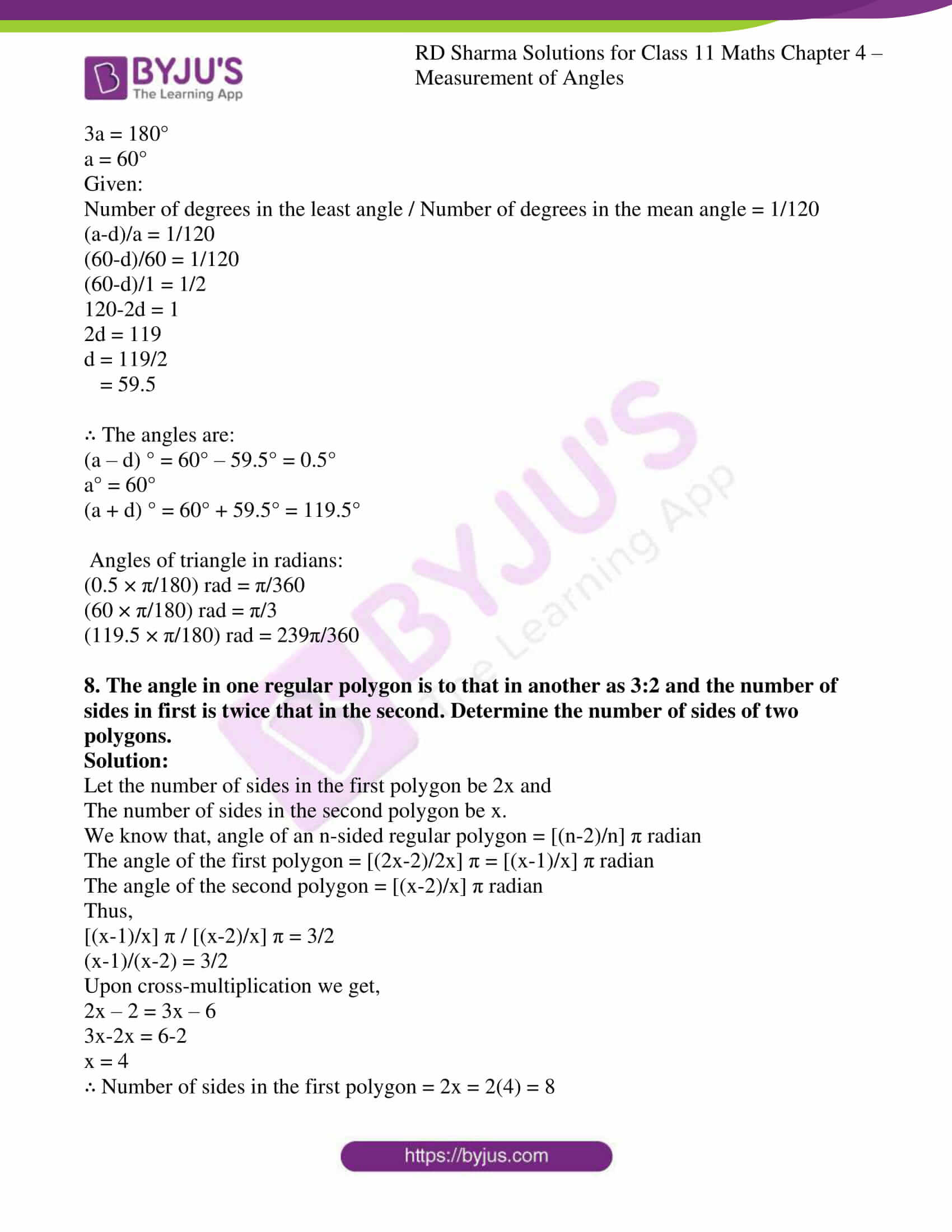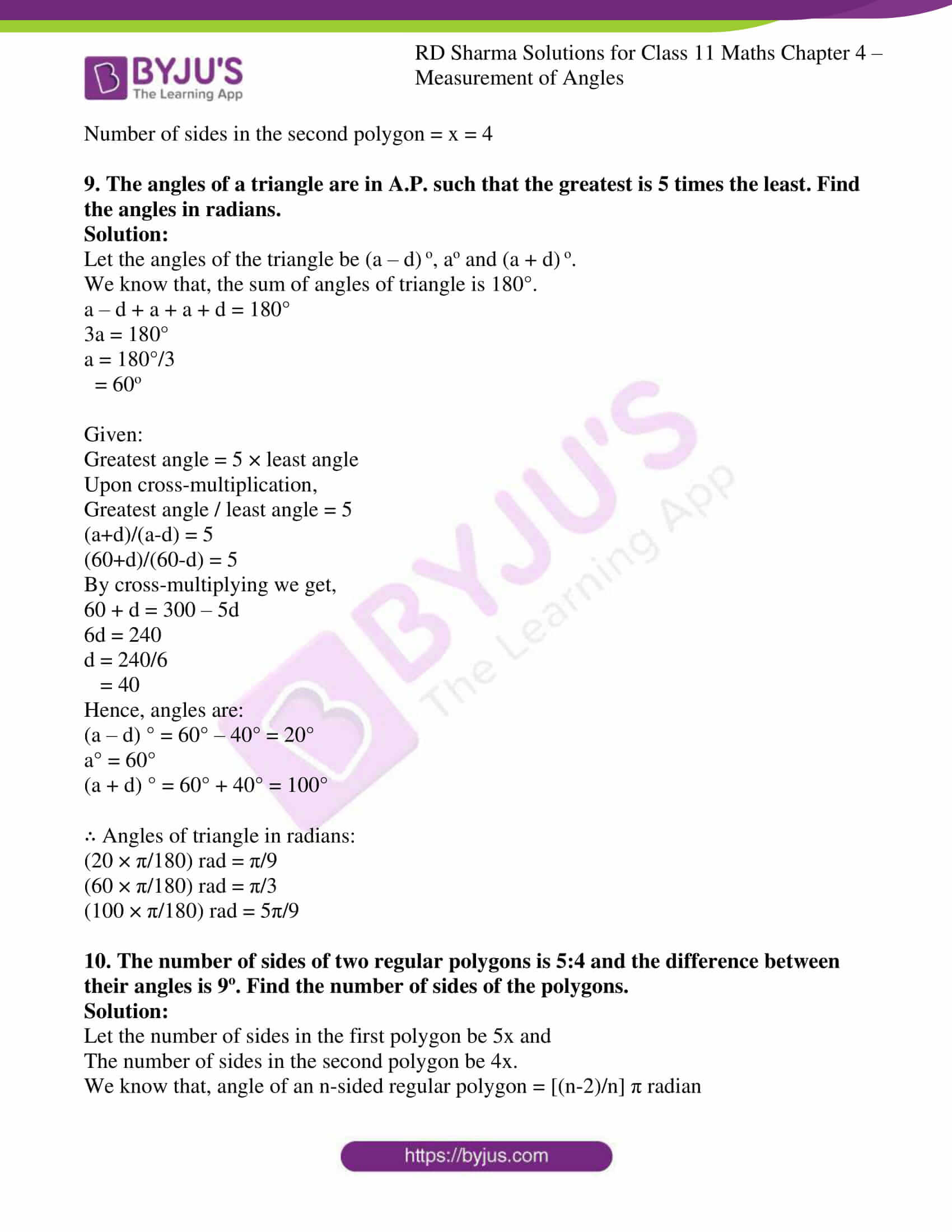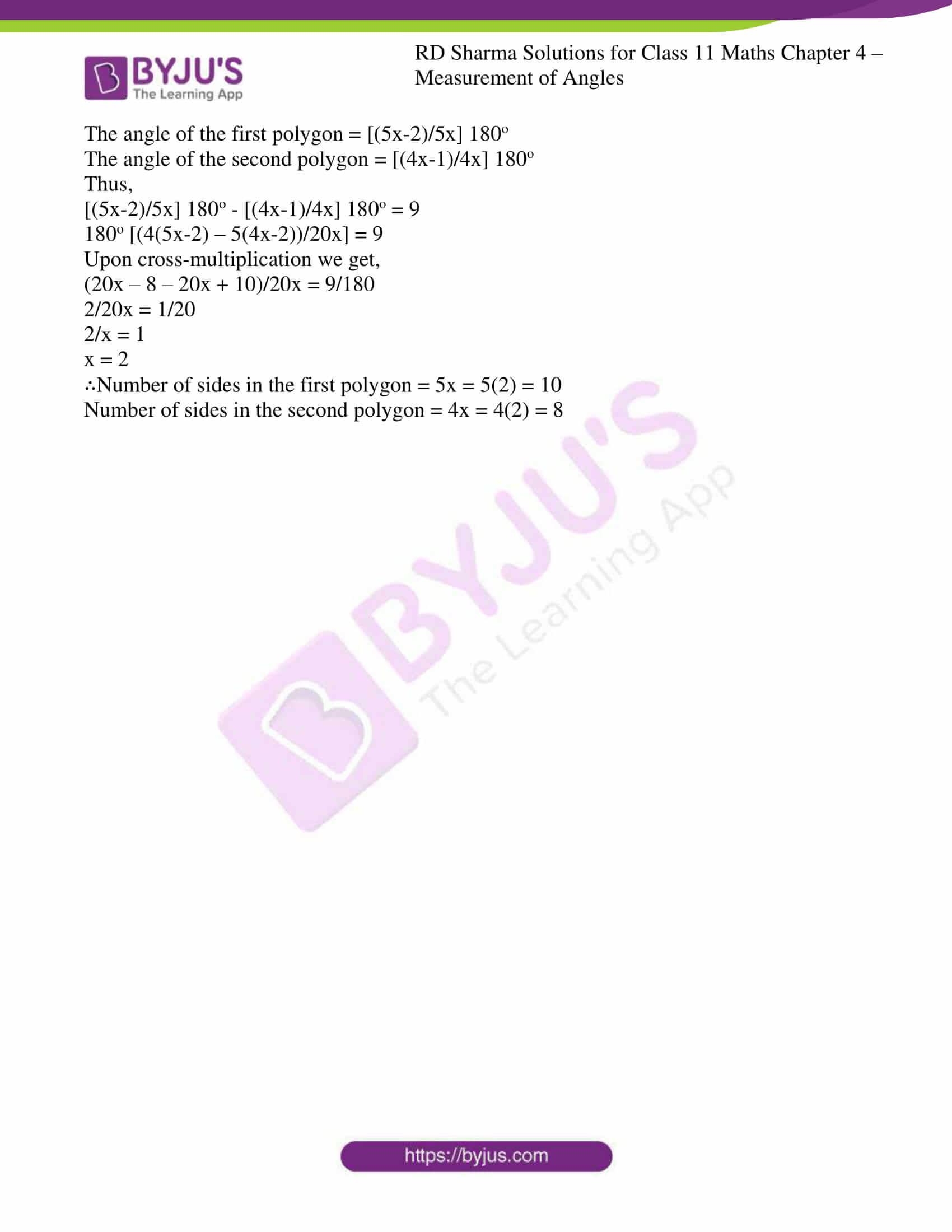### Access answers to RD Sharma Solutions for Class 11 Maths Chapter 4 – Measurement of Angles

1. Find the degree measure corresponding to the following radian measures (Use π = 22/7)
(i) 9π/5 (ii) -5π/6 (iii) (18π/5) c (iv) (-3) c (v) 11c (vi) 1c

Solution:

We know that π rad = 180° ⇒ 1 rad = 180°/ π

(i)  9π/5

[(180/π) × (9π/5)] o

Substituting the value of π = 22/7

[180/22 × 7 × 9 × 22/(7×5)]

(36 × 9) °

324°

∴ Degree measure of 9π/5 is 324°

(ii) -5π/6

[(180/π) × (-5π/6)] o

Substituting the value of π = 22/7

[180/22 × 7 × -5 × 22/(7×6) ]

(30 × -5) °

– (150) °

∴ Degree measure of -5π/6 is -150°

(iii) (18π/5)

[(180/π) × (18π/5)] o

Substituting the value of π = 22/7

[180/22 × 7 × 18 × 22/(7×5)]

(36 × 18) °

648°

∴ Degree measure of 18π/5 is 648°

(iv) (-3) c

[(180/π) × (-3)] o

Substituting the value of π = 22/7

[180/22 × 7 × -3] o

(-3780/22) o

(-171 18/22) o

(-171 o (18/22 × 60)’)

(-171o (49 1/11)’)

(-171o 49′ (1/11 × 60)’)

– (171° 49′ 5.45”)

≈ – (171° 49′ 5”)

∴ Degree measure of (-3) c is -171° 49′ 5”

(v) 11c

(180/ π × 11) o

Substituting the value of π = 22/7

(180/22 × 7 × 11) o

(90 × 7) °

630°

∴ Degree measure of 11c is 630°

(vi) 1c

(180/ π × 1) o

Substituting the value of π = 22/7

(180/22 × 7 × 1) o

(1260/22) o

(57 3/11) o

(57o (3/11 × 60)’)

(57o (16 4/11)’)

(57o 16′ (4/11 × 60)’)

(57o 16′ 21.81”)

≈ (57o 16′ 21”)

∴ Degree measure of 1c is 57o 16′ 21”

2. Find the radian measure corresponding to the following degree measures:
(i) 300o (ii) 35o (iii) -56o (iv)135o (v) -300o
(vi) 7o 30′ (vii) 125o 30’ (viii) -47o 30′

Solution:

We know that 180° = π rad ⇒ 1° = π/ 180 rad

(i) 300°

5π/3

∴ Radian measure of 300o is 5π/3

(ii) 35°

7π/36

∴ Radian measure of 35o is 7π/36

(iii) -56°

-14π/45

∴ Radian measure of -56° is -14π/45

(iv) 135°

3π/4

∴ Radian measure of 135° is 3π/4

(v) -300°

-5π/3

∴ Radian measure of -300° is -5π/3

(vi) 7° 30′

We know that, 30′ = (1/2) °

7° 30′ = (7 1/2) °

= (15/2) o

= π/24

∴ Radian measure of 7° 30′ is π/24

(vii) 125° 30′

We know that, 30′ = (1/2) °

125° 30’ = (125 1/2) °

= (251/2) o

= 251π/360

∴ Radian measure of 125° 30′ is 251π/360

(viii) -47° 30′

We know that, 30′ = (1/2) °

-47° 30’ = – (47 1/2) °

= – (95/2) o

= – (95/2 × π/180) rad

= – 19π/72

∴ Radian measure of -47° 30′ is – 19π/72

3. The difference between the two acute angles of a right-angled triangle is 2π/5 radians. Express the angles in degrees.

Solution:

Given the difference between the two acute angles of a right-angled triangle is 2π/5 radians.

We know that π rad = 180° ⇒ 1 rad = 180°/ π

Given:

2π/5

(2π/5 × 180/ π) o

Substituting the value of π = 22/7

(2×22/(7×5) × 180/22 × 7)

(2/5 × 180) °

72°

Let one acute angle be x° and the other acute angle be 90° – x°.

Then,

x° – (90° – x°) = 72°

2x° – 90° = 72°

2x° = 72° + 90°

2x° = 162°

x° = 162°/ 2

x° = 81° and

90° – x° = 90° – 81°

= 9°

∴ The angles are 81o and 9o

4. One angle of a triangle is 2/3x grades, and another is 3/2x degrees while the third is πx/75 radians. Express all the angles in degrees.

Solution:

Given:

One angle of a triangle is 2x/3 grades and another is 3x/2 degree while the third is πx/75 radians.

We know that, 1 grad = (9/10) o

2/3x grad = (9/10) (2/3x) o

= 3/5xo

We know that, π rad = 180° ⇒ 1 rad = 180°/ π

Given: πx/75

(πx/75 × 180/π) o

(12/5x) o

We know that, the sum of the angles of a triangle is 180°.

3/5xo + 3/2xo + 12/5xo = 180o

(6+15+24)/10xo = 180o

Upon cross-multiplication we get,

45xo = 180o × 10o

= 1800o

xo = 1800o/45o

= 40o

∴ The angles of the triangle are:

3/5xo = 3/5 × 40o = 24o

3/2xo = 3/2 × 40o = 60o

12/5 xo = 12/5 × 40o = 96o

5. Find the magnitude, in radians and degrees, of the interior angle of a regular:
(i) Pentagon (ii) Octagon (iii) Heptagon (iv) Duodecagon.

Solution:

We know that the sum of the interior angles of a polygon = (n – 2) π

And each angle of polygon = sum of interior angles of polygon / number of sides

Now, let us calculate the magnitude of

(i) Pentagon

Number of sides in pentagon = 5

Sum of interior angles of pentagon = (5 – 2) π = 3π

∴ Each angle of pentagon = 3π/5 × 180o/ π = 108o

(ii) Octagon

Number of sides in octagon = 8

Sum of interior angles of octagon = (8 – 2) π = 6π

∴ Each angle of octagon = 6π/8 × 180o/ π = 135o

(iii) Heptagon

Number of sides in heptagon = 7

Sum of interior angles of heptagon = (7 – 2) π = 5π

∴ Each angle of heptagon = 5π/7 × 180o/ π = 900o/7 = 128o 34′ 17”

(iv) Duodecagon

Number of sides in duodecagon = 12

Sum of interior angles of duodecagon = (12 – 2) π = 10π

∴ Each angle of duodecagon = 10π/12 × 180o/ π = 150o

6. The angles of a quadrilateral are in A.P., and the greatest angle is 120o. Express the angles in radians.

Solution:

Let the angles of quadrilateral be (a – 3d) °, (a – d) °, (a + d) ° and (a + 3d) °.

We know that, the sum of angles of a quadrilateral is 360°.

a – 3d + a – d + a + d + a + 3d = 360°

4a = 360°

a = 360/4

= 90°

Given:

The greatest angle = 120°

a + 3d = 120°

90° + 3d = 120°

3d = 120° – 90°

3d = 30°

d = 30°/3

= 10o

∴ The angles are:

(a – 3d) ° = 90° – 30° = 60°

(a – d) ° = 90° – 10° = 80°

(a + d) ° = 90° + 10° = 100°

(a + 3d) ° = 120°

(60 × π/180) rad = π/3

(80 × π/180) rad = 4π/9

(100 × π/180) rad = 5π/9

(120 × π/180) rad = 2π/3

7. The angles of a triangle are in A.P., and the number of degrees in the least angle is to the number of degrees in the mean angle as 1:120. Find the angle in radians.

Solution:

Let the angles of the triangle be (a – d) °, a° and (a + d) °.

We know that, the sum of the angles of a triangle is 180°.

a – d + a + a + d = 180°

3a = 180°

a = 60°

Given:

Number of degrees in the least angle / Number of degrees in the mean angle = 1/120

(a-d)/a = 1/120

(60-d)/60 = 1/120

(60-d)/1 = 1/2

120-2d = 1

2d = 119

d = 119/2

= 59.5

∴ The angles are:

(a – d) ° = 60° – 59.5° = 0.5°

a° = 60°

(a + d) ° = 60° + 59.5° = 119.5°

(0.5 × π/180) rad = π/360

(60 × π/180) rad = π/3

(119.5 × π/180) rad = 239π/360

8. The angle in one regular polygon is to that in another as 3:2 and the number of sides in first is twice that in the second. Determine the number of sides of two polygons.

Solution:

Let the number of sides in the first polygon be 2x and

The number of sides in the second polygon be x.

We know that, angle of an n-sided regular polygon = [(n-2)/n] π radian

The angle of the first polygon = [(2x-2)/2x] π = [(x-1)/x] π radian

The angle of the second polygon = [(x-2)/x] π radian

Thus,

[(x-1)/x] π / [(x-2)/x] π = 3/2

(x-1)/(x-2) = 3/2

Upon cross-multiplication we get,

2x – 2 = 3x – 6

3x-2x = 6-2

x = 4

∴ Number of sides in the first polygon = 2x = 2(4) = 8

Number of sides in the second polygon = x = 4

9. The angles of a triangle are in A.P. such that the greatest is 5 times the least. Find the angles in radians.

Solution:

Let the angles of the triangle be (a – d) o, ao and (a + d) o.

We know that, the sum of angles of triangle is 180°.

a – d + a + a + d = 180°

3a = 180°

a = 180°/3

= 60o

Given:

Greatest angle = 5 × least angle

Upon cross-multiplication,

Greatest angle / least angle = 5

(a+d)/(a-d) = 5

(60+d)/(60-d) = 5

By cross-multiplying we get,

60 + d = 300 – 5d

6d = 240

d = 240/6

= 40

Hence, angles are:

(a – d) ° = 60° – 40° = 20°

a° = 60°

(a + d) ° = 60° + 40° = 100°

∴ Angles of triangle in radians:

(20 × π/180) rad = π/9

(60 × π/180) rad = π/3

(100 × π/180) rad = 5π/9

10. The number of sides of two regular polygons is 5:4 and the difference between their angles is 9o. Find the number of sides of the polygons.

Solution:

Let the number of sides in the first polygon be 5x and

The number of sides in the second polygon be 4x.

We know that, angle of an n-sided regular polygon = [(n-2)/n] π radian

The angle of the first polygon = [(5x-2)/5x] 180o

The angle of the second polygon = [(4x-1)/4x] 180o

Thus,

[(5x-2)/5x] 180o – [(4x-1)/4x] 180o = 9

180o [(4(5x-2) – 5(4x-2))/20x] = 9

Upon cross-multiplication we get,

(20x – 8 – 20x + 10)/20x = 9/180

2/20x = 1/20

2/x = 1

x = 2

∴Number of sides in the first polygon = 5x = 5(2) = 10

Number of sides in the second polygon = 4x = 4(2) = 8

## Frequently Asked Questions on RD Sharma Solutions for Class 11 Maths Chapter 4

### Why should we learn all the concepts present in RD Sharma Solutions for Class 11 Maths Chapter 4?

The concepts covered in Chapter 4 of RD Sharma Solution Maths provide questions based on the exam pattern and model question paper. So it is necessary to learn all the concepts present in RD Sharma Solutions for Class 11 Maths Chapter 4. The solutions are designed based on the latest CBSE syllabus and exam pattern. Understanding the concepts before solving the exercise wise problems is very important to obtain a better score in the exam.

### List out the important topics present in RD Sharma Solutions for Class 11 Maths Chapter 4?

The topics covered in NCERT Solutions for Class 10 Maths Chapter 6 are
Angles.
Some useful terms.
Systems of measurement of angles.
Sexagesimal system.
Centesimal system.
A circular system.
The relation between degrees and radians.
The relation between radians and real numbers.
The relation between three systems of measurement of an angle.
These concepts are important from an exam perspective. It is strictly based on the latest syllabus of CBSE board, and also depends on CBSE question paper design and marking scheme.

### What are angles according to RD Sharma Solutions for Class 11 Maths Chapter 4?

An angle is formed when two rays are joined together at a common point. The common point here is called a node or vertex and the two rays are called arms of the angle. The angle is represented by the symbol ‘∠’. The word angle came from the Latin word “Angulus”. Learn more about lines and angles here. The angle is usually measured in degrees, using a protractor. Degrees 30°, 45°, 60°, 90°, 180° show different angles here. The types of angles are based on the values of angles in degrees.